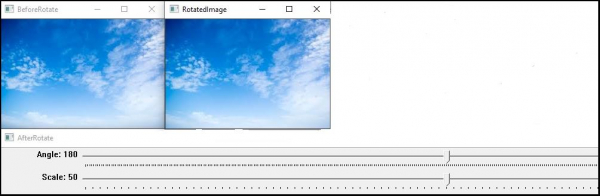# How to change the size of an image and add a border in OpenCV using C++?

In this topic, we will see another application of trackbar. Here, we will use track-bar to change the size of an image and add a border to the image and change the border's size using the track-bar.

Using the following program, we can change the size of an image, add a border, change the border's size and rotate the image. It is similar to the previous example.

The following program demonstrates how to add multiple sliders in the same track-bar.

## Example

#include<iostream>
#include<opencv2/highgui/highgui.hpp>
#include<opencv2/imgproc/imgproc.hpp>
using namespace std;
using namespace cv;
int Rotate = 180;//initializing angle//
int Scale = 50;//initializing scale//
int Border = 0;//initial Border//
Mat before_Rotate;//declaring matrix for before rotation//
int vertical = 0;//initial vertical value//
int horizontal = 0;//initial horizontal value//
void rotator(int, void*){ //function to rotate image//
Mat Rotation = getRotationMatrix2D(Point(horizontal, vertical),(Rotate - 180), Scale / 50.0);//affine transformation matrix for 2D rotation//
Mat Rotated;//matrix for rotated image
warpAffine(before_Rotate, Rotated, Rotation, before_Rotate.size(), INTER_LINEAR, Border, Scalar());//applying affine transformation//
imshow("RotatedImage", Rotated);//show rotated image//
}
int main(int argc,char**argv) {
vertical = before_Rotate.rows / 2;//getting midpoint of vertical pixels//
horizontal = before_Rotate.cols / 2;//getting midpoints of horizontal pixels//
namedWindow("BeforeRotate");//declaring window to show image before rotation//
imshow("BeforeRotate", before_Rotate);//showing image before rotation//
namedWindow("AfterRotate");//declaring window to show image after rotation//
createTrackbar("Angle", "AfterRotate", &Rotate, 360, rotator);//creating trackbar for rotation//
createTrackbar("Scale", "AfterRotate", &Scale, 100, rotator);//creating trackbar to change size//
createTrackbar("Border Mode", "After Rotate", &Border, 5, rotator);//creating trackbar to add border//
int cbfunction = 0;//initiate value of rotator function's argument//
rotator(cbfunction, &cbfunction);//call back rotator function//
waitKey(0);//wait till keystroke//
return 0;
}

## Output